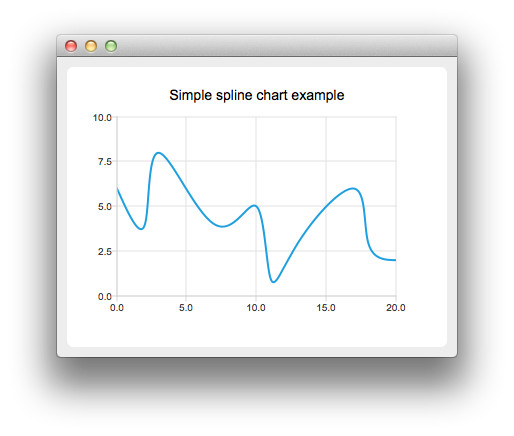# Creating Spline ChartsTo create spline charts we need to put our data into QSplineSeries. QSplineSeries automatically calculates spline segment control points that are needed to properly draw the spline.

```auto series = new QSplineSeries;
series->setName("Spline");```

Now let's add some data points to the series.

```series->append(0, 6);
series->append(2, 4);
series->append(3, 8);
series->append(7, 4);
series->append(10, 5);
*series << QPointF(11, 1) << QPointF(13, 3) << QPointF(17, 6) << QPointF(18, 3) << QPointF(20, 2);```

The data series has been populated. To display it on a chart we create a QChart object and add the data series to it. We also set the title and the values range on the y-axis, so that our chart's visibility is better.

```auto chart = new QChart;
chart->legend()->hide();
`createDefaultChartView(chart);`